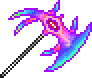•类型 \l!--\n --\g\{\{#vardefine:_typepage\!\{\{#or:\{\{l10n\!item_infobox\!typepage:@@@@\}\}\!\{\{tr\!\{\{ucfirst:@@@@\}\}\!link\ey\}\}\}\}\}\}\l!--\_\n --\g\{\{#vardefine:_typetext\!\{\{#or:\{\{l10n\!item_infobox\!type:@@@@\}\}\!\{\{tr\!\{\{ucfirst:@@@@\}\}\}\}\}\}\}\}\l!--\_\n --\g\{\{nowrap\!class\etag\!\{\{ifexists\!\{\{#var:_typepage\}\}\!\(\(\{\{#var:_typepage\}\}\!\{\{#var:_typetext\}\}\)\)\!\{\{#var:_typetext\}\}\}\}\}\}\l!--\n --\g 55 8.75（很强击退力） 36（很慢速度）3

## 花絮

• 此武器参考了《Soul Eater（噬魂师）》动画系列中的一个特殊攻击，具体地说是鬼神狩（Kishin Hunter）的 Maka Albarn 的变体，这可能也是把它移除掉的原因。（译注：指版权方面的原因）
• 这是三把可能由于参考了外部品牌而从电脑版中移除的武器之一。